NEET  >  Short & Long Answer Questions: Gravitation - 1

# Short & Long Answer Questions: Gravitation - 1 | Physics Class 11 - NEET

 1 Crore+ students have signed up on EduRev. Have you?

Q.1 Why is the law of gravitation called the universal law?

Answer: This is because of the fact that the law of gravitation holds good for any pair of bodies in the universe whether microscopically small or astronomically large in size.
Q.2 Why is G called the universal gravitational constant?

Answer: This is because the value of G is same for any pair of the bodies in the universe. It does not depend on the nature of the medium between the two bodies or on the nature of the bodies themselves.
Q.3 What is the ratio of the force of attraction between two bodies kept in air and the same distance apart in water?

Answer: 1:1, because the gravitational force does not depend on the nature of the medium.
Q.4 If spheres of same material and same radius r are touching each other, then show that the gravitational force between them is directly proportional to r4.

Answer: If ρis the density of the material, then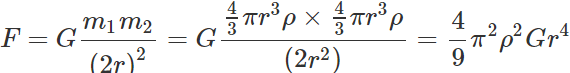Clearly, F∝r4

Q.5 If the density of planet is doubled without any change in its radius, how does g change on the planet?

Answer: It gets doubled, because g∝ρ.
Q.6 Is it possible to shield a body from gravitational effects?

Answer: No, it is not possible to shield a body from gravitational effects because gravitational interaction does not depend upon the nature of the intervening medium.
Q.7 If the force of gravity acts on all bodies in proportion to their masses, why does a heavy body not fall faster than a light body?

Answer: If F be the gravitational force on a body of mass m, then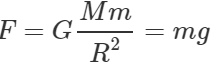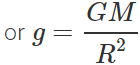Clearly, F∝m m but g does not depend on m. Hence all bodies fall with same rapidness if there is no air resistance.
Q.8 The mass of the moon is nearly 10% of the mass of the earth. What will be the gravitational force of the earth on the moon, in comparison to the gravitational force of the moon on the earth?

Answer: Both forces will be equal in magnitude as gravitational force between two bodies is a mutual force.
Q.9 Earth is continuously pulling the moon towards its centre, still it does not fall to the earth. Why?

Answer: Gravitational force of attraction due to earth provides the centripetal force, which keeps the moon in orbit around the earth. Moreover, this gravitational force acts perpendicular to the velocity of the moon.
Q.10 We cannot move finger without disturbing all stars. Why?

Answer: When we move our finger, the distance between the objects and our finger changes. Hence, the force of attraction changes, disturbing the entire universe, including the stars.
Q.11 According to Newton's law of gravitation, the apple and the earth experience equal and opposite forces due to gravitation. But it is the apple that falls towards the earth and not vice-versa. Why?

Answer: According to Newton's third law of motion, the force with which the earth is attracted towards the apple is equal to the force with which earth attracts the apple. However, the mass of the earth is extremely large as compared to that of apple. So acceleration of the earth is very small and is not noticeable.
Q.12 According to Newton's law of gravitation, every particle of matter attracts every other particle. But bodies on the surface of earth never move towards each other on account of this force of attraction. Why?

Answer: Because of their small masses, the gravitational attraction between two bodies is too small to produce any motion in them. But due to the large mass of the earth, the gravitational attraction between the bodies and earth is very large and so all bodies are attracted towards the centre of the earth.
Q.13 Does the gravitational force of attraction of the earth become zero at some height above the earth? Give reason.

Answer:  No, the gravitational force of attraction of earth on a body at height h is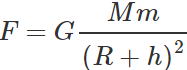Clearly, F will be zero only when h is infinity.
Q.14 Which is more fundamental-mass or weight of a body?

Answer: Mass is more fundamental than weight because the mass of a body remains constant while its weight changes from place to place due to change in the value of g.
Q.15 If the diameter of the earth becomes twice its present value but its mass remains unchanged, then how would be the weight of an object on the surface of the earth affected?

Answer: Weight of body,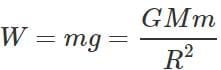When the diameter or radius of the earth becomes double its present value, the weight of the body will be W′=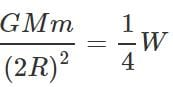i.e., weight will become one-fourth of the present value.
Q.16 If the diameter of the earth becomes half its present value but its average density remains unchanged then how would be the weight of an object on the surface of the earth affected?

Answer: Acceleration due to gravity on the surface of the earth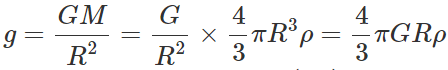When the diameter or radius becomes half its present value,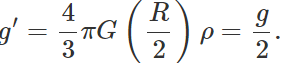Hence the weight of the object will be halved.
Q.17 The mass and diameter of a planet are twice those of the earth. What will be the time-period of that pendulum on this planet, which is a second's pendulum on the earth?

Answer:  Initially, g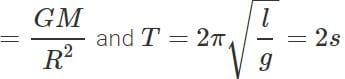For a second's pendulum)          When M and R are doubled, g′=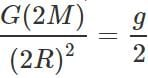and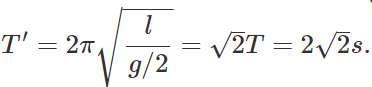Q.18 If the radii of two planets be R1 and R2 and their mean densities be ρ1 and ρ2, then prove that the ratio of accelerations due to gravity on the planets will be R1ρ1:R2ρ2.

Answer: On the surface of any planet, g=4/3 πGRρ ∴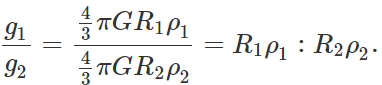Q.19 The distance between two bodies A and B is r. Taking the gravitational force according to the law of inverse square of r, the acceleration of the body is a. If the gravitational force follows an inverse fourth power law, then what would be the acceleration of the body A?

Answer: For inverse square law,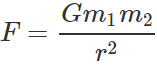∴  Acceleration of body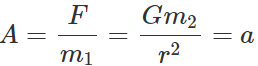For inverse fourth power law, F′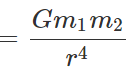Acceleration of body A=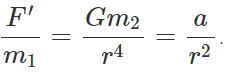Q.20 Why is centre of mass of a body often called its centre of gravity?

Answer: The torque due to gravity on a body acts as if its entire mass were concentrated at its centre of mass. That is why centre of mass of a body is often called its centre of gravity.
Q.21 Why the value of g is more at the poles than at the equator?

Answer: As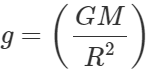and the value of R at equator is greater than that at poles, hence g at poles is greater than g at equator.
Q.22 Why a body weighs more at the poles than at the equator?

Q.23 Where does a body weigh more? At the sea level or on the mountains?

Answer: At the sea level because weight decreases with altitude.
Q.24 Where will a body weigh more - 1 km above the surface of earth or 1 km below the surface of earth?

Answer: As gh=g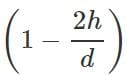and gd=g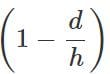For h=d=1km,clearly gd>gp. Hence the body will weigh more at a depth of 1 km below the surface of earth.
Q.25 Does the concentration of the earth's mass near its centre change the variation of g with height compared with a homogeneous sphere? How?

Answer: Any change in the distribution of the earth's mass will not affect the variation of acceleration due to gravity with height. This is because for a point outside the earth, the whole mass of the earth is 'effective? and the earth behaves as a homogeneous sphere.
Q.26 The weight of a body is less inside the earth than on the surface. Why?

Answer: As we go inside the earth, the value of the attracting mass M decreases and hence the value of g decreases. Therefore, the weight of the body mg is less inside the earth than on the surface.
Q.27 Why do you feel giddy while moving on a merry-go-round?

Answer: When moving in a merry-go-round, our weight appears to decrease when we move down and appears to increase when we move up.
Q.28 At which place on earth's surface, the value of g is largest and why?

Answer: The value of g is largest at the poles due to following two reasons: (a) Distance of poles from the centre of earth is smaller than the distance of any other point on earth's surface from its centre. (b) At poles, no centrifugal force acts on the body.
Q.29 When dropped from the same height a body reaches the ground quicker at poles than at the equator. Why?

Answer: The acceleration due to gravity is more at the poles than at the equator. When the initial velocities and distances travelled are the same, the time taken by the body is smaller if the acceleration due to gravity is large. Hence, when dropped from the same height, a body reaches the ground quicker at the poles than at the equator.

Q.30 When a clock controlled by a pendulum is taken from the plains to a mountain, it becomes slow but a wrist-watch controlled by a spring remains unaffected. Explain the reason for the difference in the behaviour of the two watches.

Answer: Due to decrease in the value of g at the mountain, the time period of the pendulum of the clock increases. On the other hand, the spring watch remains unaffected by the variation.
Q.31 A clock fitted with a pendulum and another with a spring indicate correct time on earth. Which shows correct time on the moon?

Answer: A clock fitted with a spring will show correct time on the moon, because its time period is not affected by the variation.
Q.32 Why does a tennis ball bounce higher on a hill than on plains?

Answer: The value of g is less on hills because they are comparatively at a greater distance from the centre of the earth. Therefore, the gravitational pull on the tennis ball is less on hill tops and so it bounces higher on hills than on plains.
Q.33 A man can jump six times as high on the moon as that on the earth. Justify. Or Explain, why one can jump higher on the surface of the moon than that on the earth.

Answer: The value of ′g′ on the surface of moon is about 1/6 th of its value on the surface of earth. For the same gain of P.E. in both cases, we have mgmhm=mgehe or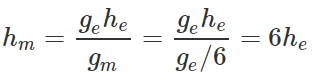Q.34 Moon-travellers tie heavy weight at their back before landing on the moon. Why?

Answer: Due to the small value of g on the moon, the traveller would weigh less. To compensate for this loss in weight, the travellers load their backs with heavy weight.
Q.35 Why is earth flat at the poles?

Q.36 What is the effect of rotation of the earth on the acceleration due to gravity?

Answer: The acceleration due to gravity decreases due to rotation of the earth. This effect is zero at the poles and maximum at the equator.
Q.37 Name two factors which determine whether a planet would have an atmosphere or not.

Answer: The two such factors are (i) The value of acceleration due to gravity on the planet. (ii) Surface temperature of the planet.
Q.38 If the earth stops rotating about its axis, what will be the effect on the value of g? Will this effect be same at all places?

Answer: The value of g will increase at all places except at the poles. The increase will be different at different places, maximum at the equator.
Q.39 If the earth stops rotating about its axis, then by what value will the acceleration due to gravity change at the equator?

Answer: The value of g increases by ω2R
Q.40 Explain why tidal waves (high tide and low tide) are formed on seas.

Answer: The gravitational attraction of moon on sea water causes high tides. Tides at one place cause low tides (ebbs) at another. Attraction by sun also causes tides but only of half the magnitude. Hence on new moon and full moon days, when both effects add, tides are very high.
Q.41 Why are we not thrown off the surface of the earth by the centrifugal force?

Answer: The force of gravity exerted on our body by the earth towards its centre is greater than centrifugal force acting on our body away from the centre.
Q.42 A satellite does not need any fuel to circle around the earth. Why?

Answer: The gravitational force between satellite and earth provides the centripetal force required by the satellite to move in a circular orbit.
Q.43 Why a multi-stage rocket is required to launch a satellite?

Answer: The multistage rocket helps to economize the consumption of fuel.
Q.44 Why do different planets have different escape velocities?

Answer: As υe=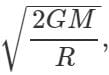therefore escape velocities have different values on different planets which are of different masses and different sizes.
Q.45 An elephant and an ant are to be projected out of earth into space. Do we need different velocities to do so?

Answer: The escape velocity,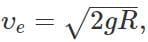, does not depend upon the mass of the projected body. Thus we need the same velocity to project an elephant and an ant into space.
Q.46 Does a rocket really need the escape velocity of 11.2 kms−1 initially to escape from the earth?

Answer: No, rocket can have any velocity at the start. The rocket can continue to increase the velocity due to thrust provided by the escaping gases that will carry it to a desired position.
Q.47 The moon has no atmosphere. Why?

Answer: Due to the small value of ′g′, the escape velocity on the moon surface is small(2.38 kms-1). The air molecules have thermal velocities greater than the escape velocity. Therefore, the air molecules escape away and cannot form atmosphere on the moon.
Q.48 The earth has atmosphere. Why?

Answer: Because the r. m. s. velocity of air molecules is less than escape velocity on the earth, the air cannot escape from the surface of the earth. Hence the earth has atmosphere.
Q.49 Lighter gases like H2, He, etc. are rare in the atmosphere of the earth. Why?

Answer: Usually the average velocity of the molecules of lighter gases is greater than the escape velocity at the earth's surface. So lighter gases have escaped from the earth's atmosphere.
Q.50 The gravitational force exerted by the sun on the moon is greater than (about twice as great as) the gravitational force exerted by the earth on the moon. Why then doesn't the moon escape from the earth (during a solar eclipse, for example)?

Answer: The moon can escape only if the moon has no orbital motion. In fact, while revolving around the earth, the moon has orbital motion around the sun also. The gravitational attraction of the sun on the moon provides the centripetal force required for the orbital motion around the sun.

The document Short & Long Answer Questions: Gravitation - 1 | Physics Class 11 - NEET is a part of the NEET Course Physics Class 11.
All you need of NEET at this link: NEET

## Physics Class 11

124 videos|464 docs|210 tests

## Physics Class 11

124 videos|464 docs|210 tests

### How to Prepare for NEET

Read our guide to prepare for NEET which is created by Toppers & the best Teachers

Track your progress, build streaks, highlight & save important lessons and more!(Scan QR code)

,

,

,

,

,

,

,

,

,

,

,

,

,

,

,

,

,

,

,

,

,

;